## 【Flag】机器学习100天-[第1天]-数据清洗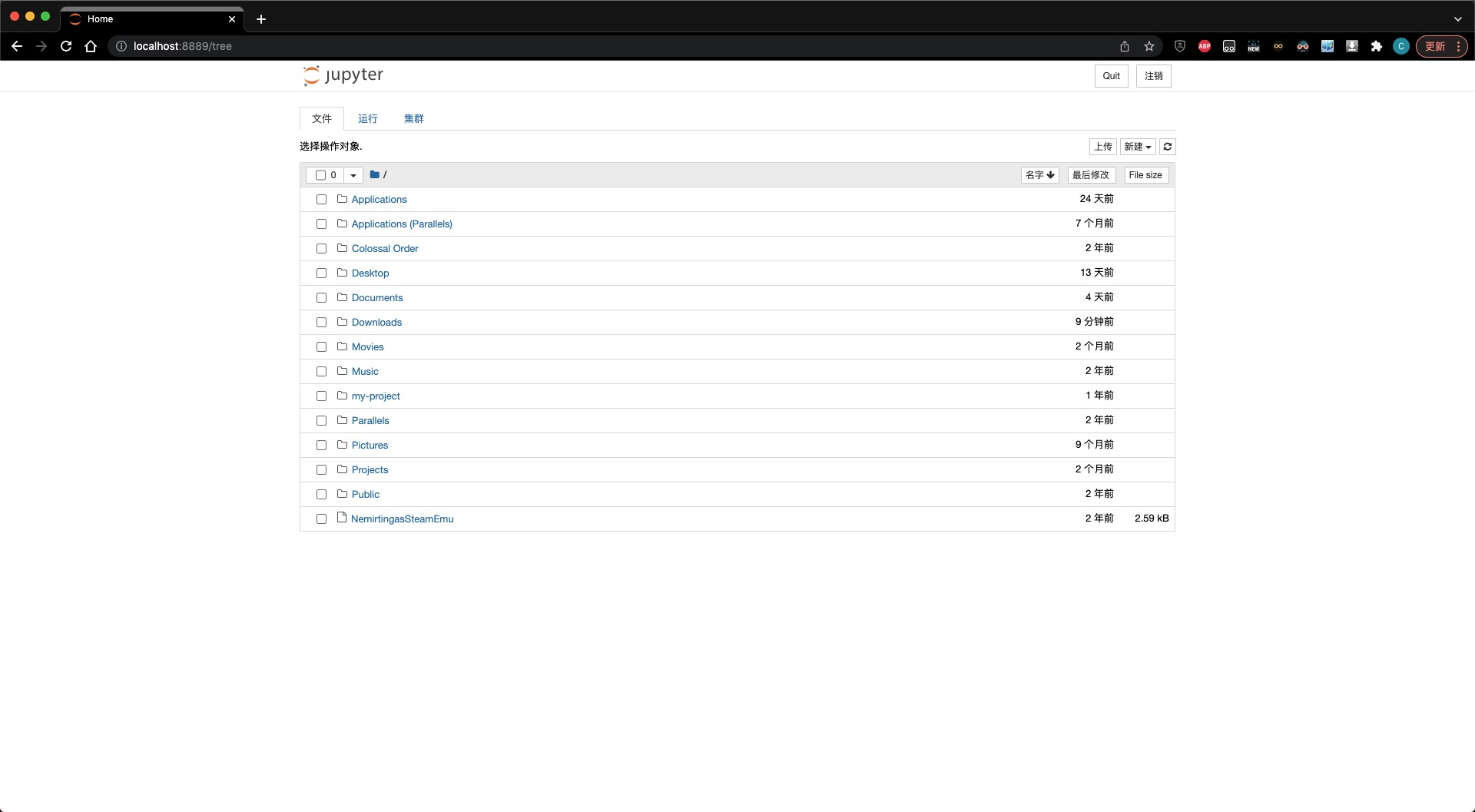## 数据预处理的基本步骤

1. 导入数据处理相关库：其中最常见的库是 numpy 以及 pandas 。其他的库可以根据需要再进行导入；
3. 处理丢失数据：如遇到数据集不完整的情况，为不影响模型的性能，或纯粹为了模型能够跑起来，我们需要处理数据中的空值(或其他类型的非数值)，并用整列的均值、中值、众数或特定的常数进行处理。完成这一步骤我们可以使用 sklearn.impute 中导入SimpleImputer 方法来进行处理；
4. 解析分类数据(Encoding categorical data)：分类数据指的是还有标签值而不是数字的变量(如定序、定类以及定距变量等，或其他的非数字标签)。这类型的数据通常是固定的，如原始数据中的 purchased 向量中的 YES 和 NO。但这些标签是无法进行数学计算的，因此我们要根据一定的规则，将其转换为数字。通常，我们可以通过 sklearn.preprocessing 库中的 LabelEncoder 类 来完成这一步骤的处理；
5. 将数据集拆分为训练集和测试集：其中训练集用于训练模型。训练好后，再用测试集来验证模型预测的结果。一般来说，这两者的数据量比为 —-> 训练集:测试集 = 80:20 。而拆分数据集可以我们可以通过 sklearn.crossvalidation 中的tran_test_split()方法实现。
6. 特征缩放(Feature Scaling)：大部分模型算法在计算两点间的距离时一般用欧式距离。但此特特征在幅度、单位以及范围上变化很大。在距离计算时，高幅度的特征比低幅度的特征权重更大。为此，可使用特征标准或Z值归一化将所有数据映射到[0，1] 或 [-1, 1]区间内，避免”距离”数量级差距过大的影响。(参考:这里)

## 创建项目目录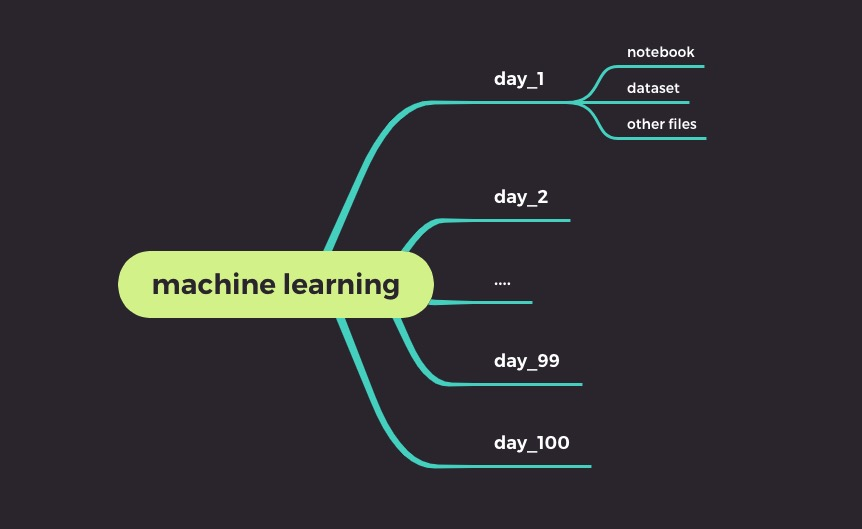day_1文件目录

## 查看原始数据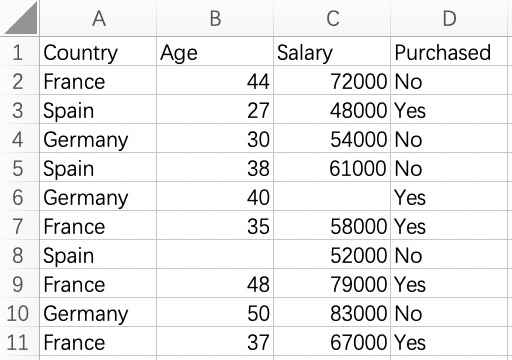## 开始编码

### 导入库和数据集

import numpy as np
import pandas as pd

X = dataset.iloc[ : , :-1].values
Y = dataset.iloc[ : , 3].values

### 处理丢失数据

from sklearn.impute import SimpleImputer as Imputer
imputer = Imputer(missing_values = np.NAN, strategy = "mean")
imputer = imputer.fit(X[ : , 1:3])
X[ : , 1:3] = imputer.transform(X[ : , 1:3])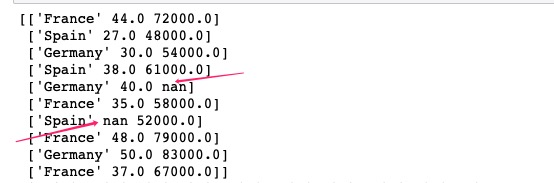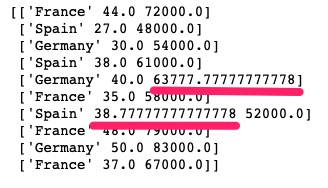The imputation strategy. If “mean”, then replace missing values using the mean along the axis. 使用平均值代替

If “median”, then replace missing values using the median along the axis.使用中值代替

If “most_frequent”, then replace missing using the most frequent value along the axis.使用众数代替，也就是出现次数最多的数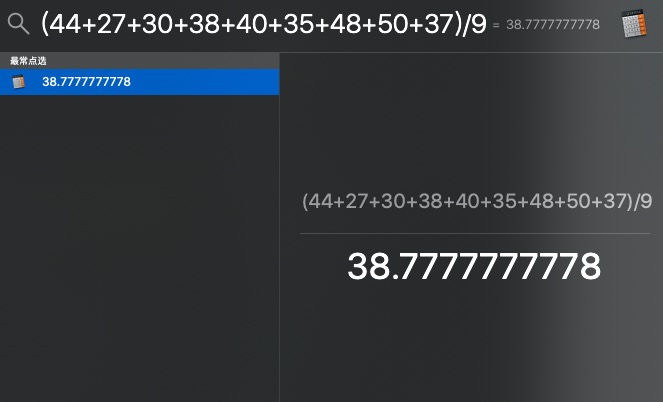SI = SimpleImputer(missing_values=np.NAN, strategy=’mean’)

I = Imputer(missing_values=’NaN’, strategy=’mean’, axis=0, verbose=0, copy=True)

### 解析分类数据

from sklearn.preprocessing import LabelEncoder, OneHotEncoder
labelencoder_X = LabelEncoder()
X[ : , 0] = labelencoder_X.fit_transform(X[ : , 0])

print(X[ : , 0])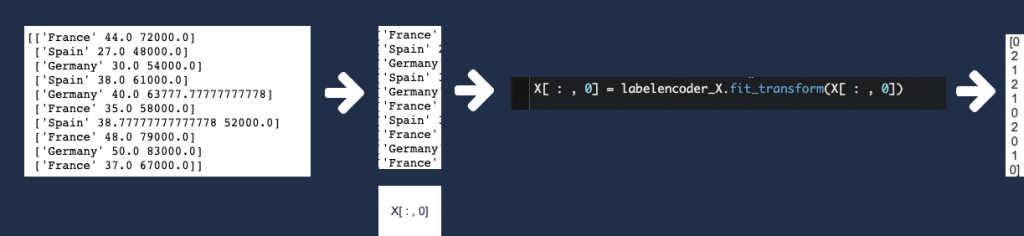### 创建哑变量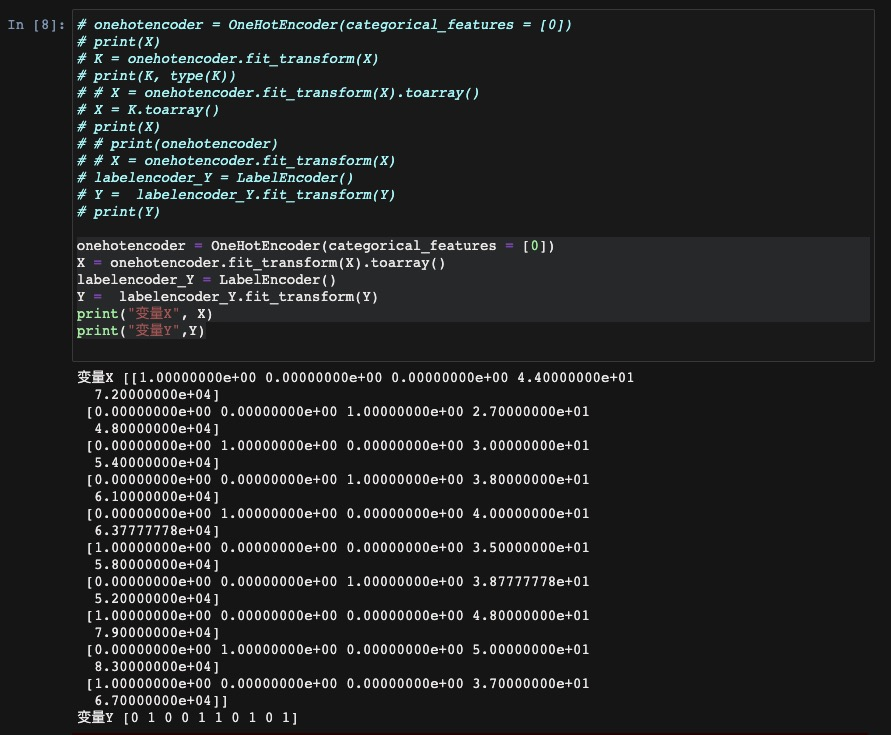onehotencoder = OneHotEncoder(categorical_features = )
X = onehotencoder.fit_transform(X).toarray()
labelencoder_Y = LabelEncoder()
Y = labelencoder_Y.fit_transform(Y)
print("变量X", X)
print("变量Y",Y)

### 拆分数据集为训练集合和测试集合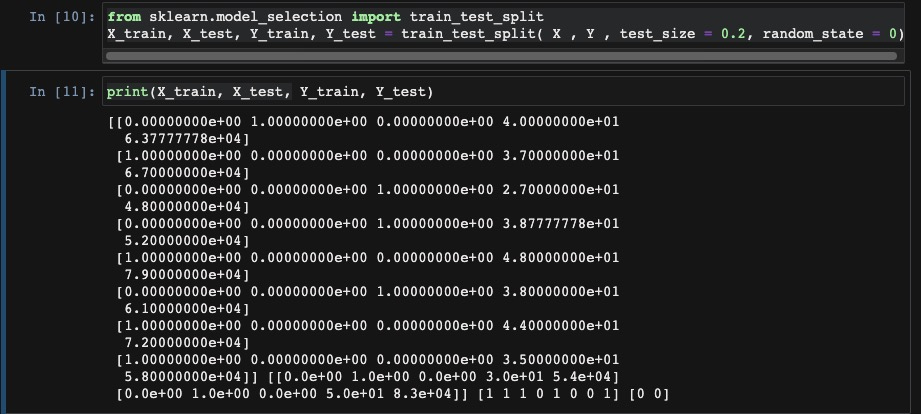</span> </span>

from sklearn.model_selection import train_test_split
X_train, X_test, Y_train, Y_test = train_test_split( X , Y , test_size = 0.2, random_state = 0)

### 特征缩放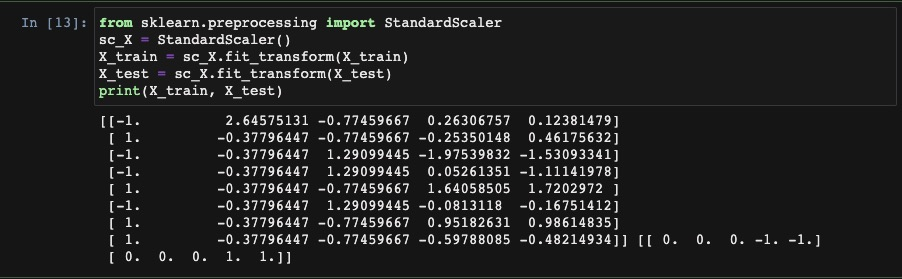from sklearn.preprocessing import StandardScaler
sc_X = StandardScaler()
X_train = sc_X.fit_transform(X_train)
X_test = sc_X.fit_transform(X_test)
print(X_train, X_test)

## 相关知识补充

1. 进行归一化操作的原因，以及常用的归一化方法可参考本文
2. fit(), fit_transform(), transform()在不同方法下的异同可参考本文

## 笔记下载

文件名称：笔记文件and数据集
文件大小：1MB
下载声明：本站部分资源来自于网络收集，若侵犯了你的隐私或版权，请及时联系我们删除有关信息。
下载地址：Data, day_1.ipynb